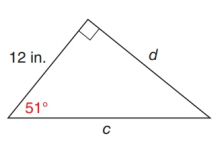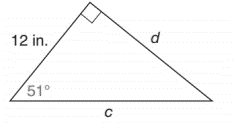Chapter 11.2, Problem 21EElementary Geometry For College St...

7th Edition
Alexander + 2 others
ISBN: 9781337614085

Solutions

Chapter
SectionElementary Geometry For College St...

7th Edition
Alexander + 2 others
ISBN: 9781337614085
Textbook Problem

In Exercise 17 to 22, use either the sine ratio or the cosine ratio to find the length of the indicated sides of the triangle correct to the nearest tenth of a unit.To determine

To find:

The length of the indicated sides of the following triangle,Explanation

Consider the following figure,

General formula for cosine ratio and sin ratio is given below,

From the given figure the value c is the hypotenuse value since it is opposite to right angle.

Then 12 in. is the length of the leg adjacent to 51 and d is the length of leg opposite to 51.

Therefore,

cos51=12cc=12cos51

Using a scientific calculator in degree mode, follow the following key sequence,

51cos0

Still sussing out bartleby?

Check out a sample textbook solution.

See a sample solution

The Solution to Your Study Problems

Bartleby provides explanations to thousands of textbook problems written by our experts, many with advanced degrees!

Get Started

Evaluate each expression: 35+6853252

Elementary Technical Mathematics

What are the two requirements for a random sample?

Statistics for The Behavioral Sciences (MindTap Course List)

If f(x) = 2e3x, f(x) = a) 2e3x b) 2xe3x c) 6e3x d) 6xe3x

Study Guide for Stewart's Single Variable Calculus: Early Transcendentals, 8th